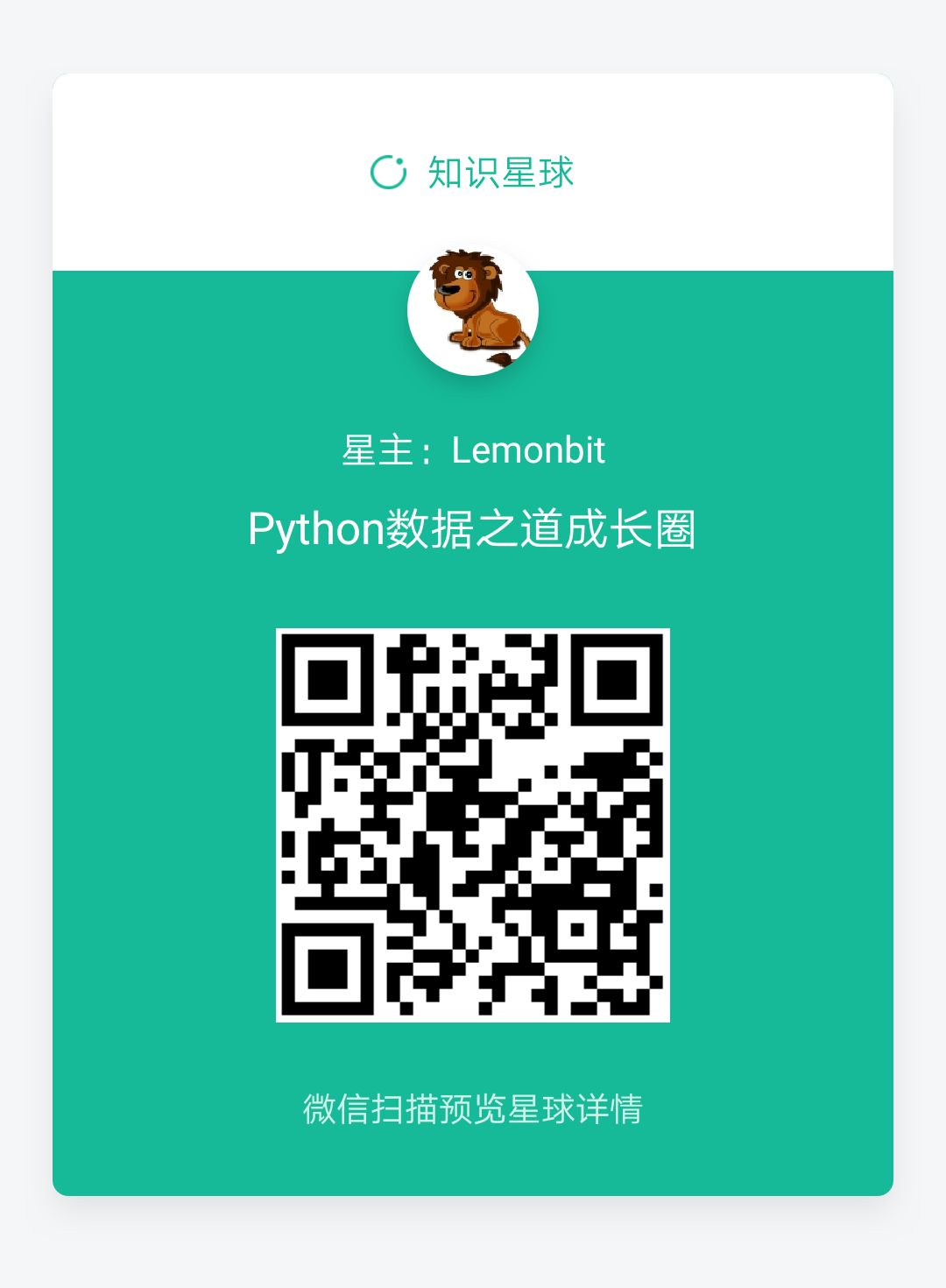• # weight_data 的数据类型为 dict def random_weight(weight_data): total = sum(weight_data.values()) # 权重求和 ra = random.uniform(0, total) # 在0与权重和之前获取一个随机数 curr_sum = 0 ...

1 随机抽奖

本次运行环境为：win7

jupyter notebook

python 3.6

1.1 随机抽取一个元素

用 random.choice() 来实现随机抽取一个元素。from random import choice

import random

import pandas as pd

name = ['张三', '李四', '王五', '小明', '小郑', '梅西', 'C罗', '内少']

random_name = choice(name)

random_name

1.2 随机抽取多个元素

可以考虑用 random.sample() 来随机抽取多个元素。# 从name中随机选择3个元素

slice_sample = random.sample(name, 3)

slice_sample

1.3 考虑权重后随机抽取一个元素

如果是不同的成员有不同的权重，比如本次抽奖，在赞赏的同时有评论的人员，赋予的权重要求稍微高一些。可以自定义一个函数，用来考虑权重后随机抽取一个元素。# weight_data 的数据类型为 dict

def random_weight(weight_data):

total = sum(weight_data.values())    # 权重求和

ra = random.uniform(0, total)   # 在0与权重和之前获取一个随机数

curr_sum = 0

ret = None

#     keys = weight_data.iterkeys()    # 使用Python2.x中的iterkeys

keys = weight_data.keys()        # 使用Python3.x中的keys

for k in keys:

curr_sum += weight_data[k]             # 在遍历中，累加当前权重值

if ra <= curr_sum:          # 当随机数<=当前权重和时，返回权重key

ret = k

break

return ret

# 用Pandas从excel中读取数据

# 将数据进行整理

df_data = df[['name', 'weight']].set_index('name')

# 根据上面的上述，获取dict类型的数据

data = df_data.to_dict()['weight']

random_weight(data)

1.4 考虑权重后，随机获取多个元素

最终，本次需要在考虑权重后，随机获取多个元素(N个)，并且不能重复，可以在上个函数的基础上，再添加一个自定义函数，来一次性加权随机抽取多个元素。def choujiang(data, N):

#     N = 6

results = []

i = 0

while i

m = random_weight(data)

if m in results:

continue

results.append(m)

i = i+1

return results

choujiang(data,3)

---------------- End ----------------

点击前往【项目实战】

知识星球

我的知识星球【Python数据之道成长圈】已开通，想加入的同学，请回复数字 “2” 了解详情。展开全文python加权求和
• 这是一个非常快速的完全矢量化版本：def vectorized(prob_matrix, items):s = prob_matrix.cumsum(axis... r).sum(axis=0)return items[k]理论上,搜索排序是用于在累积求和概率中查找随机值的正确函数,但是当m相对较...

这是一个非常快速的完全矢量化版本：

def vectorized(prob_matrix, items):

s = prob_matrix.cumsum(axis=0)

r = np.random.rand(prob_matrix.shape)

k = (s < r).sum(axis=0)

return items[k]

理论上,搜索排序是用于在累积求和概率中查找随机值的正确函数,但是当m相对较小时,k =(s

In : %timeit original(prob_matrix, items)

1 loops, best of 3: 270 ms per loop

In : %timeit improved(prob_matrix, items)

10 loops, best of 3: 24.9 ms per loop

In : %timeit vectorized(prob_matrix, items)

1000 loops, best of 3: 1 ms per loop

定义函数的完整脚本是：

import numpy as np

def improved(prob_matrix, items):

# transpose here for better data locality later

cdf = np.cumsum(prob_matrix.T, axis=1)

# random numbers are expensive, so we'll get all of them at once

ridx = np.random.random(size=n)

# the one loop we can't avoid, made as simple as possible

idx = np.zeros(n, dtype=int)

for i, r in enumerate(ridx):

idx[i] = np.searchsorted(cdf[i], r)

# fancy indexing all at once is faster than indexing in a loop

return items[idx]

def original(prob_matrix, items):

choices = np.zeros((n,))

# This is slow, because of the loop in Python

for i in range(n):

choices[i] = np.random.choice(items, p=prob_matrix[:,i])

return choices

def vectorized(prob_matrix, items):

s = prob_matrix.cumsum(axis=0)

r = np.random.rand(prob_matrix.shape)

k = (s < r).sum(axis=0)

return items[k]

m = 10

n = 10000 # Or some very large number

items = np.arange(m)

prob_weights = np.random.rand(m, n)

prob_matrix = prob_weights / prob_weights.sum(axis=0, keepdims=True)

展开全文python加权求和
• 节目小主持人选拔赛，选手的最终得分不是评分直接加总，...首先，我们用一个常见的excel加权求和函数来做。公式为：=SUMPRODUCT(B2:B9,C2:C9)。函数是Excel的灵魂，的确没错。求乘积之和，Sumproduct函数用得最多。...

节目小主持人选拔赛，选手的最终得分不是评分直接加总，而是要先乘以各自的权重之后再求和。Excel数据源如下：excel计算加权总分的应用其实蛮常见的，各自使用的方法也不尽相同，用操作技巧、函数公式等等都可以实现。

首先，我们用一个常见的excel加权求和函数来做。公式为：=SUMPRODUCT(B2:B9,C2:C9)。

函数是Excel的灵魂，的确没错。求乘积之和，Sumproduct函数用得最多。Sumproduct这个数组函数，如果大量使用，Excel表格会变得超级卡。

雪儿突然想到了滴答老师在《Excel极速贯通班》讲到过超级表非常强大，花了70多分钟来讲解“超级表”应用。

下面我们来初识超级表的面貌。

第一步，随便选中一个有数据源的任意单元格，执行“插入——表格”命令。第二步，“创建表”数据源会默认选中A1：C9单元格区域。确定。第三步，此时，效果如下所示。第四步，在D1单元格输入“加权分数”字样。D2单元格输入公式：=B2*C2，此时，Excel会自动进行每一行的计算。截图如下：第五步，在“设计”标签，勾选“汇总行”。Excel会自动得到加权总分。使用超级表得到的结果，和使用Sumproduct函数得到的结果一样。

展开全文• 使用加权总和工具可以对多个输入进行加权及组合，以创建整合式分析。它可以轻松地将多个栅格输入(代表多种因素)与组合权重或相对重要性相结合，在这一方面它与加权叠加工具很相似。这两种工具有两个主要区别：加权...

使用加权总和工具可以对多个输入进行加权及组合，以创建整合式分析。它可以轻松地将多个栅格输入(代表多种因素)与组合权重或相对重要性相结合，在这一方面它与加权叠加工具很相似。

这两种工具有两个主要区别：

加权总和工具不能将重分类值重设为评估等级。

加权总和工具允许使用浮点型和整型值作为输入，而加权叠加工具只接受整型栅格作为输入。

不将重分类值重设到评估等级，分析可保持其分辨率。例如，在适宜性模型中，如果有 10 个重新分类到 1 至 10 等级的输入条件(10 为最佳)，并且未对其指定权重，则加权总和输出值的范围可以从 10 到 100。对于同样的输入，加权叠加将把 10 至 100 的重分类分析范围规范化至评估等级，如返回到 1 至 10 等级。当只需要识别少数几个最适合的位置或指定数量的地点时，在加权总和中保持模型分辨率会很有用。

一般来说，连续栅格的值会分为不同的类别。例如，不同的坡度值可以分为平坦、适中、陡峭和非常陡峭。每个坡度值都可以指定为这些类别之一，并且指定了重分类值的类别相对于叠加分析中的条件，可以更好地表明该类的倾向性。使用重分类工具可以对这些栅格进行重新分类。

加权叠加工具最常用于适宜性建模，并且可用于确保遵循正确的方法。加权总和工具在需要保持模型分辨率，或需要浮点型输出或小数权重时很有用。

加权总和可将每个输入栅格的指定字段值与指定权重相乘。然后将所有输入栅格相加来创建输出栅格。

运行加权总和工具的步骤如下：

添加栅格。单击输入栅格箭头再单击输入栅格，或浏览到输入栅格再单击添加。栅格即被添加到“加权总和”表中。重复此过程来输入下一个栅格，依次类推。

选择字段。对于每一个输入栅格，单击字段列来指定将要在叠加分析中使用的字段。将出现一个下拉列表显示所有可用于输入的字段。该字段必须为数值字段。

为输入栅格指定权重。可基于每个输入栅格的重要性对其进行加权，或为其指定一个影响百分比。对于每一个输入栅格，单击权重行来指定值。该权重可以是任意浮点型值(包括负值)。

运行此工具。将每个输入栅格的像元值与其栅格权重相乘。将结果像元值相加即会生成最终输出栅格。

转载自：https://blog.csdn.net/dsac1/article/details/38063947

展开全文• 作者认为在计算原型时应该为每个样本赋予相应的权重，再进行加权求和得到该类别对应的原型，这样得到的原型就是所谓的本质特征了。本文提出的网络处理过程如下图所示 ??首先，支持集图像和查询集图像都要经过一个...
• 摘要使用经常使用測量比例叠加多个栅格数据，并依据各栅格数据的重要性分配权重。插图插图中，两个输入栅格已又一次分类为 1 至 3 三种公共測量级别。为每一个栅格均分配了一个影响百分比。...由于加权...
• 一、地理加权回归模型概述橘生淮南则为橘，生于淮北则为枳，叶徒相似，其实味不同。所以然者何？水土异也。——《晏子春秋·内篇杂下》这段文字很好的描述了空间异质性。从地理空间的角度，经济发展尤其是区域经济...
• import java.util.HashMap; import java.util.Map; import java.util.Random; public class Test1 { // String 可以为任意类型 也可以自定义类型 static Map keyChanceMap = new HashMap(); static { // ...
• 加权中位数定义如下：如果x是N个元素的排序数组,并且w是总权重W的权重数组,则加权中值是最后的x [i],使得w [i]和所有先前权重之和小于大于或等于S / 2.在C中,可以这样表示(假设x,w和W如上所定义)double sum = 0;int ...c语言加权成绩
• 给定一个嵌套的整数列表，返回列表中所有整数的总和，并按其深度加权。每个元素可以是整数，也可以是列表-其元素也可以是整数或其他列表。与之前的问题从根到叶的权重增加的问题不同，现在从下至上定义了权重。也...
• How do I go about calculating weighted mean of a Map where the Integer value is the weight for the Double value to be averaged.eg: Map has following elements:(0.7, 100) // value is 0.7 and weight is 1...java 加权平均
• QGIS中的加权质心计算 QGIS Processing Toolbox 附带了一个名为的内置工具Mean Coordinate，可用于从点图层计算加权中心。 首先，让我们计算几何质心。加载karnataka图层并启动处理工具箱 → 质心算法。 将结果...
• 最近需要一种自定义loss，可以对每个实例的loss进行不同的加权。在网上找到的代码，没有我想要的，因此首先对torch的loss进行了研究。torch的loss有包装在nn.Module之中的，有在nn.functional之中的，两种的区别就是...loss 加权
• public class mymy{ public static void main(String[] args) { //main 方法 //调用class的sum方法 mymy.sum(10,29); int i=30; mymy.sum(i,30); } public static void sum(int a,int b){ System.... } } 方法，求和java
• 我目前正在使用以下查询来获取一些数字： SELECT gid, count(gid), (SELECT cou FROM size WHERE ...-------------------------------+ 我试图计算加权平均,即 (1*19+4*27+1*556)/(19+27+556) 有没有办法使用单个查询？mysql 加权
• 我在arm上通过neon的函数来优化矩阵所有点求和，参考的资料是《DEN0018A_neon_programmers_guide》 neon优化后的矩阵所有点求和为0.16ms，opencv使用sum函数的求和时间为0.8ms。下面是我的代码，具体的函数表示可以...
• 输入一个嵌套列表，嵌套层次不限，根据层次，求列表元素的加权和。第一层每个元素 的值为：元素值*1，第二层每个元素的值为：元素值*2，第三层每个元素的值为：元素值*3， ...,以此类推！ 输入格式: 在一行中输入...python
• 通常小学、初中的成绩单里出现的平均分数都不需要加权，甚至也不需要用到函数，高中以上可能是因为考试制度的关系，有时候会需要用到加权分数，例如要上的大学比较重视的科目，利用「SUMPRODUCT」可以计算出加权后的...
• Java：多个数求和

千次阅读 2021-02-26 20:53:09java多个数求和
• 有可能，但真的很复杂：np.random.seed(234)df= pd.DataFrame(np.random.randint(5,8,(1000,4)), columns=['a','b','c','d'])wm = lambda x: (x * df.loc[x.index, "c"]).sum() / x.sum()wm.__name__ = 'wa'f = ...
• 矩阵求和 3.1 爱因斯坦求和 对指定维度求和 A= torch.zeros((2, 4)) #形状为2*4 B= torch.zeros((2, 4)) #形状为2*4 sum = torch.einsum("bs,bs->b",A,B) #形状为2，第二个维度做内积求和操作 4 .矩阵拼接 4.1 水平...pytorch 深度学习 神经网络
• 该楼层疑似违规已被系统折叠隐藏此楼查看此...想对每j列进行对应项求和相加，整了一上午都……谢谢大家！B(:,:,1,1)=1.0e+010*9.69302.1590-4.08422.15902.2769-1.7028-4.0842-1.70283.0083B(:,:,2,1)=1.0e+010*9.69...
• 也是让我们理解，为什么最近9年（2012-2021），人类最厉害的人工智能算法卷积神经网络，其实只是计算加权平均。 理解卷积的词义 卷积是英文convolution的翻译，convolution的英文释义是盘绕，弯曲（a twist or curve...卷积 卷积神经网络 机器学习
• 阈值逻辑单元阈值逻辑单元(threshold logic unit ，TLU)，它可以输入一组加权系数的量，对它们进行求和，如果这个和达到或者超过了某个阈值，输出一个量。例如，输入为a1, a2, ..., an和权值 w1, w2,..., wn。接着是...
• 本文介绍Attention机制，其经典使用是在NLP中搭配RNN（一般为LSTM）做编码解码器（Seq2Seq），用于给编码过程中每一步的状态分配权重，然后加权求和得到解码过程中每一步解码时的状态向量，参与计算，得到本步的输出...神经网络 自然语言处理 深度学习
• {"moduleinfo":{"card_count":[{"count_phone":1,"count":1}],"search_count":[{"count_phone":4,"count":4}]},"card":[{"des":"阿里技术人对外发布原创技术内容的最大平台；社区覆盖了云计算、大数据、人工智能、...
• 而 loss_weights 是要用在加权求和中，得到一个整体的 loss，然后用同一个 optimizer 对神经网络的参数进行更新。 (更新）最近尝试训练一个字体风格转换程序，对不同的任务使用不同的 optimizer，发现在初期确实有效...loss 加权
• Pandas 窗口计算

千次阅读 2021-01-14 15:05:22
查看更新日志Pandas 窗口函数，为了处理数字数据，Pandas 提供几种窗口函数，如移动窗口函数(rolling())，扩展窗口函数(expanding())，指数加权滑动(ewm())，同时可在基基础上调用适合的统计函数，如求和、中位数、...
• 传送门：理解生成对抗网络（GAN） 第二阶段：归一化处理 得到了相似度si，进行归一化处理就得到了权重系数，一般使用softmax函数： 第三阶段：计算注意力 最后一步就是对权重系数进行加权求和： 带注意力机制的自...深度学习 自然语言处理 java 人工智能 python
• #include <iostream> #include <string> #include <string.h> #include <vector>... int a = { 7,9,10,5,8,4,2,1,6,3,7,9,10,5,8,4,2 };... char b = { '1','0', 'X',....